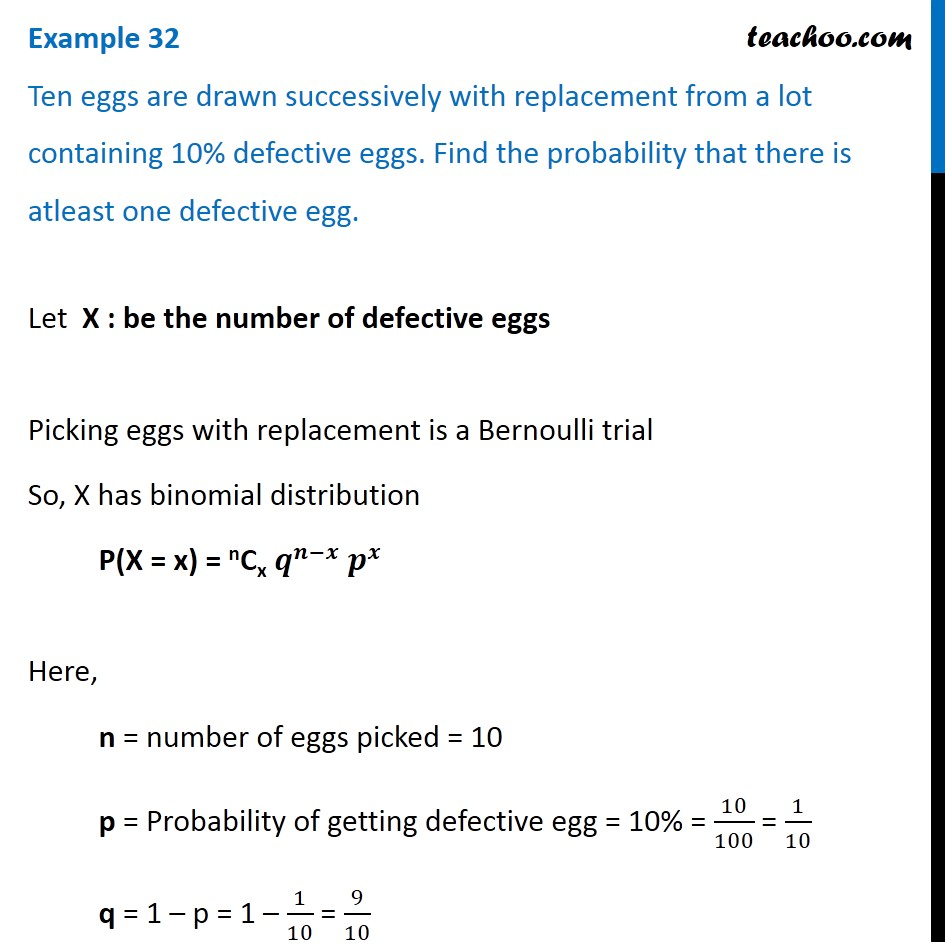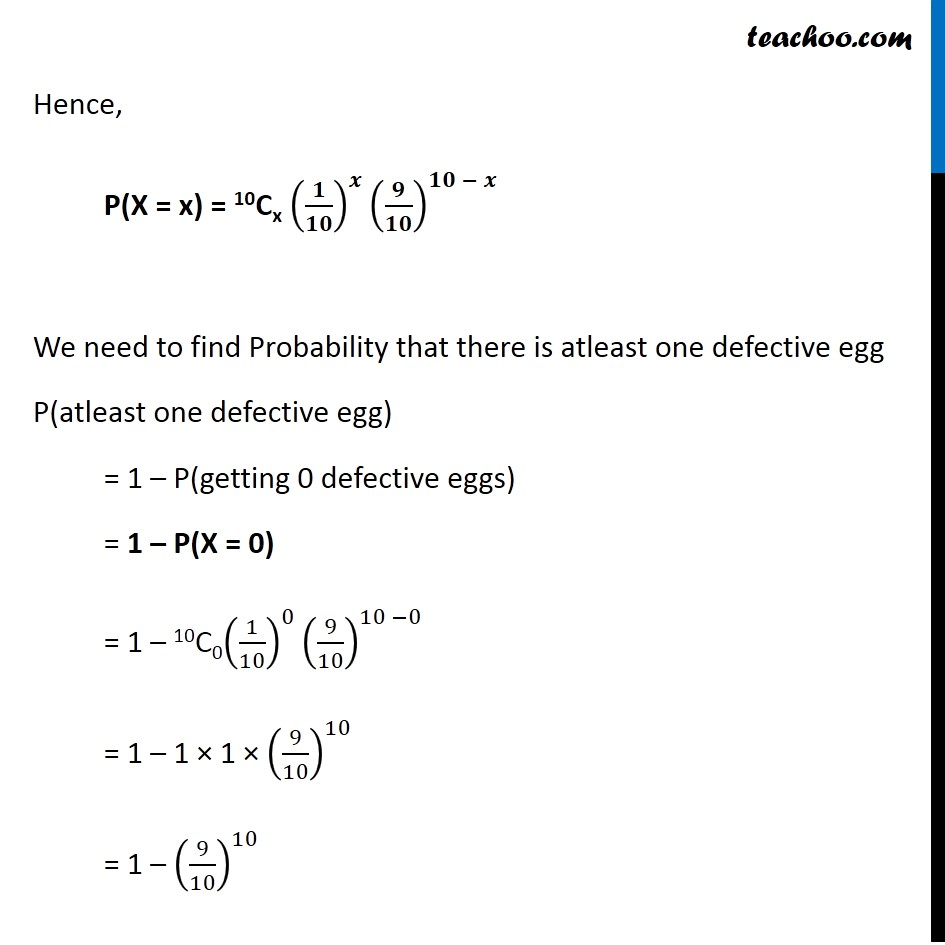Chapter 13 Class 12 Probability

Class 12
Important Questions for exams Class 12Learn in your speed, with individual attention - Teachoo Maths 1-on-1 Class

### Transcript

Question 11 Ten eggs are drawn successively with replacement from a lot containing 10% defective eggs. Find the probability that there is atleast one defective egg.Let X : be the number of defective eggs Picking eggs with replacement is a Bernoulli trial So, X has binomial distribution P(X = x) = nCx 𝒒^(𝒏−𝒙) 𝒑^𝒙 Here, n = number of eggs picked = 10 p = Probability of getting defective egg = 10% = 10/100 = 1/10 q = 1 – p = 1 – 1/10 = 9/10 Hence, P(X = x) = 10Cx (𝟏/𝟏𝟎)^𝒙 (𝟗/𝟏𝟎)^(𝟏𝟎 − 𝒙) We need to find Probability that there is atleast one defective egg P(atleast one defective egg) = 1 – P(getting 0 defective eggs) = 1 – P(X = 0) = 1 – 10C0(1/10)^0 (9/10)^(10 −0) = 1 – 1 × 1 × (9/10)^10 = 1 – (9/10)^10